# Generalised Impulse Response Functions

Ken Nyholm, 22 March 2016

Generalised impulse response functions (GIRFs) are calculated from the moving average representation of the VAR model, as the difference between the a conditional and unconditional forecast, where the conditioning information set is the shock to the j'th variable (koop et al (1996)).

Let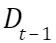represent the data available at time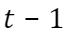, let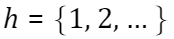be the forecast horizon, let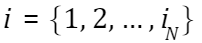count the variables in included in the VAR model, which are collected in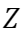, such that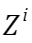refers to the i'th variable, and denote by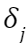the shock to the j'th variable in the VAR, where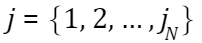, with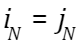.

The GIRF is then defined in the following way: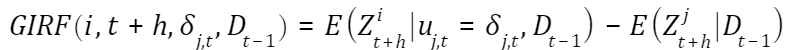for the VAR model: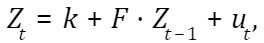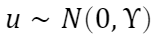Following Peseran and Shin (1998) the orthogonalised and generalised IRFs can be calculated in the following way, respectively, for a shock to the j'th equation: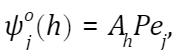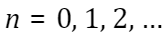where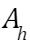is the h'th coefficient-matrix from the moveing average representation of the VAR model, P is the lower-triangular Cholesky decomposition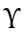,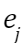is a vector having unity at the j'th position and zeros elsewhere, and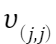is the (j,j) element of.

The VAR model is written here as a VAR(1) model without loss of generality due to the companion form.

For illustrative purposes, three calculation methods are applied:

1. Orthogonalised IRFs via cholesky factorisation
2. GIRFs via conditional forecasting
3. GIRFs implemented via the formulas in Peseran and shin

I use US and EA yield curve data, and set-up the VAR model using Nelson-Siegel yield curve fators, i.e. the level, slope and curvature. The loaded data are observed daily, but for the analysis I sample every 20th observation, to mimic monthly data, and there

obs_freq = (1:20:length(Dat_tot))';

dates_ = x2mdate(Dat_tot(obs_freq,1));

US = Dat_tot(obs_freq,[2:12]);

EA = Dat_tot(obs_freq,[13:23]);

x_space = (1:20:length(dates_))';

lambda = 0.06;

tau = [3 12:12:120]';

nTau = length(tau);

H = [ones(nTau,1) (1-exp(-lambda.*tau))./(lambda.*tau) ...

(1-exp(-lambda.*tau))./(lambda.*tau)-exp(-lambda.*tau) ];

figure

plot(H)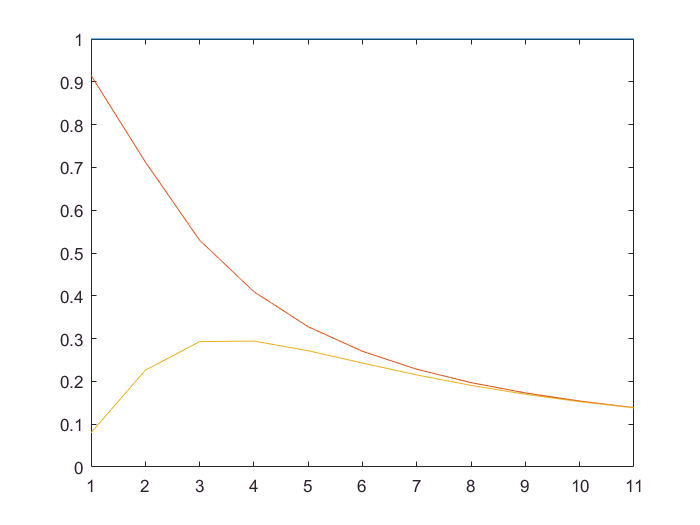F_US = US*pinv(H)';

F_EA = EA*pinv(H)';

figure

plot(F_US), title('US Yield Curve Factors')

set(gca,'XTick', x_space, 'XTicklabel',datestr(dates_(x_space,1),'mm/yy'))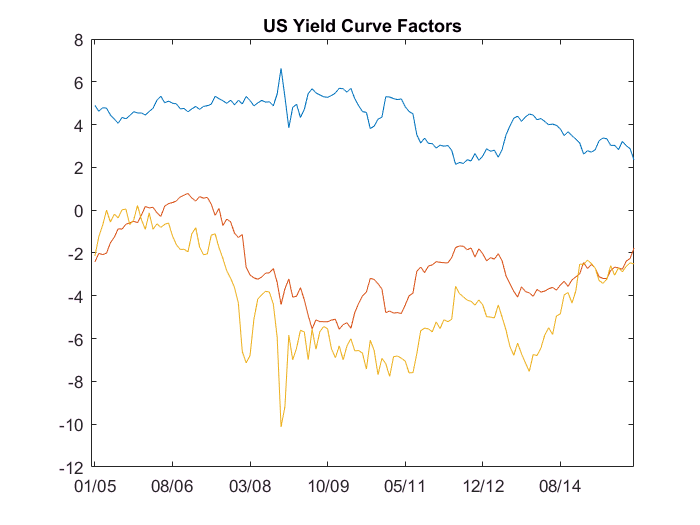figure

plot(F_EA), title('EA Yield Curve Factors')

set(gca,'XTick', x_space, 'XTicklabel',datestr(dates_(x_space,1),'mm/yy'))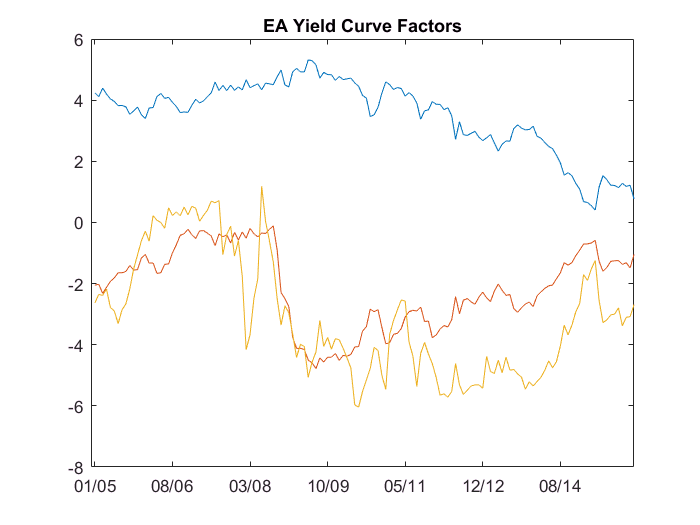The optimal lag-length of the VAR is determined using the AIC and BIC criterions. The maximal tested lag-length is 10 days.

calc_Dat = [F_US F_EA];

[nObs,nVars] = size(calc_Dat);

lags_ = 10; % max lag-length tested

opti_Lag = zeros(lags_,5);

for ( j = 1:lags_ )

Model_1 = [];

Est_Model1 = [];

Model_1 = vgxset('n', nVars, 'nAR', j, 'Constant', true);

[Est_Model1, Est_StdErrs1, LLF1, W1] = vgxvarx( Model_1, calc_Dat );

[NumParam, NumActive] = vgxcount( Est_Model1 );

[isStable, isInvertible] = vgxqual( Est_Model1 );

[AIC,BIC] = aicbic(LLF1, NumActive, nObs);

opti_Lag(j,:) = [ j, AIC, BIC, isStable, isInvertible ];

end

disp(' ')

disp(' ... Optimal Lag length... ')

... Optimal Lag length...

disp(' Lag AIC BIC isStable isInvertible')

Lag      AIC        BIC     isStable   isInvertible

disp(opti_Lag)

1.0e+03 *

0.0010    0.5294    0.7147    0.0010    0.0010
0.0020    0.5276    0.8188    0.0010    0.0010
0.0030    0.5213    0.9184    0.0010    0.0010
0.0040    0.5376    1.0406    0.0010    0.0010
0.0050    0.5345    1.1434    0.0010    0.0010
0.0060    0.5519    1.2667    0.0010    0.0010
0.0070    0.5625    1.3832    0.0010    0.0010
0.0080    0.5720    1.4987    0.0010    0.0010
0.0090    0.5581    1.5906    0.0010    0.0010
0.0100    0.5264    1.6648    0.0010    0.0010

opt_AIC = find(opti_Lag(:,2)==min(opti_Lag(:,2)))

opt_AIC = 3

opt_BIC = find(opti_Lag(:,3)==min(opti_Lag(:,3)))

opt_BIC = 1

Relying on the BIC criterion, the optimal lag-length is found to be 2, and it is observed that all estimated models are stable and invertible.

In order to calculate the IRFs, the VAR model is converted into its moving-average representation. This facilitates the calculation of the IRFs following Peseran and Shin. Below, as an alternative way to calculate the generalised IRFs, calculations are done following a direct implementation of the above definition of the GIRFs as the difference between conditional and unconditional projections from the model, using as conditioning information consecutive shocks to each of the variables included in the VAR model. Incidently, this is also the way the impulse response functions are described in Matlab's help function to the econometric toolbox.

EstSpec = vgxset('n', nVars, 'nAR', opt_BIC, 'Constant', true);

[Est_M, Est_Std, LLF, W_est] = vgxvarx( EstSpec, calc_Dat(opt_BIC+1:end,:),...

[],calc_Dat(1:opt_BIC,:));

% ... Convert the VAR into MA representation with nMAlags number of lags

%

nMA_lags = 20;

SpecMA = vgxma(Est_M, nMA_lags, 1:nMA_lags );

SpecMA.MA(2:nMA_lags+1) = SpecMA.MA(1:nMA_lags);

SpecMA.MA{1} = eye(nVars);

% ... IRFs: IRF_o = orthogonalised

% IRF_g = generalised

%

IRF_o = zeros(nMA_lags,nVars,nVars); % [1:nlags, 1:nvariables, shocked variable ]

IRF_g = zeros(nMA_lags,nVars,nVars);

IRF_g1 = zeros(nMA_lags,nVars,nVars);

P = chol(SpecMA.Q,'lower');

sig_jj = diag(SpecMA.Q);

for ( j=1:nVars )

indx_ = zeros(nVars,1);

indx_(j,1) = 1;

for ( k=1:nMA_lags ) % k counts the lag

IRF_o(k,:,j) = SpecMA.MA{k}*P*indx_; % Peseran-Shin eqn 7

IRF_g1(k,:,j) = SpecMA.MA{k}*SpecMA.Q*indx_;

IRF_g(k,:,j) = sig_jj(j,1).^(-0.5).*IRF_g1(k,:,j); % Peseran-Shin eqn 10

end

end

% ... alternative GIRF using the direct definition as the difference between

% a conditional and unconditional projection (as also seen

% in the matlab help/doc)

%

IRF_gAlt = zeros(nMA_lags,nVars,nVars);

W0 = zeros(nMA_lags, nVars); % Innovations without a shock

for ( j=1:nVars )

W1 = W0;

%W1(1,:) = P(:,j)'; % Generates Orthogonalised IRFs

%W1(1,:) = IRF_g(1,:,j);

een = zeros(6,1);

W = zeros(6,1);

een(j,1) = 1;

W(j,1) = sqrt(SpecMA.Q(j,j));

tmp = SpecMA.Q*een.*SpecMA.Q(j,j)^(-1)*W';

W1(1,:) = tmp(:,j)';

F_cond = vgxproc( Est_M, W1); % Conditional Projection (with shock)

F_ = vgxproc( Est_M, W0); % Unconditional Projection (without shock)

IRF_gAlt(:,:,j) = (F_cond-F_);

end

Visual comparisons between the calculated IRFs are shown below for each variable, i.e. for the level, slope, and curvature of the yield curve in the US and EA areas.

for ( j=1:6 )

shk_var = j;

yLab = ['Shock to var#: ' num2str(j)];

figure

subplot(3,2,1), plot([ squeeze(IRF_o(:,1,shk_var)) ...

squeeze(IRF_g(:,1,shk_var)) ...

squeeze(IRF_gAlt(:,1,shk_var)) ]),

legend('O','G','G_{alt}'), title('US L'),

ylabel(yLab)

subplot(3,2,2), plot([ squeeze(IRF_o(:,2,shk_var)) ...

squeeze(IRF_g(:,2,shk_var)) ...

squeeze(IRF_gAlt(:,2,shk_var)) ]),

legend('O','G','G_{alt}'), title('US S')

ylabel(yLab)

subplot(3,2,3), plot([ squeeze(IRF_o(:,3,shk_var)) ...

squeeze(IRF_g(:,3,shk_var)) ...

squeeze(IRF_gAlt(:,3,shk_var)) ]),

legend('O','G','G_{alt}'), title('US C')

ylabel(yLab)

subplot(3,2,4), plot([ squeeze(IRF_o(:,4,shk_var)) ...

squeeze(IRF_g(:,4,shk_var)) ...

squeeze(IRF_gAlt(:,4,shk_var)) ]),

legend('O','G','G_{alt}'), title('EA L')

ylabel(yLab)

subplot(3,2,5), plot([ squeeze(IRF_o(:,5,shk_var)) ...

squeeze(IRF_g(:,5,shk_var)) ...

squeeze(IRF_gAlt(:,5,shk_var)) ]),

legend('O','G','G_{alt}'), title('EA S')

ylabel(yLab)

subplot(3,2,6), plot([ squeeze(IRF_o(:,6,shk_var)) ...

squeeze(IRF_g(:,6,shk_var)) ...

squeeze(IRF_gAlt(:,6,shk_var)) ]),

legend('O','G','G_{alt}'), title('US C')

ylabel(yLab)

end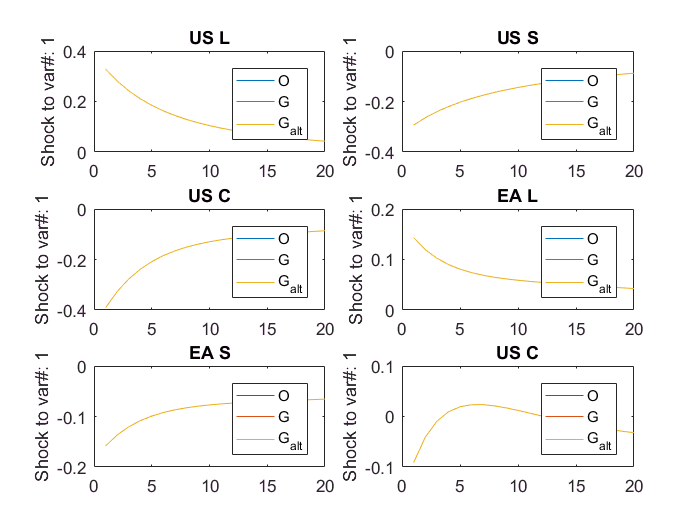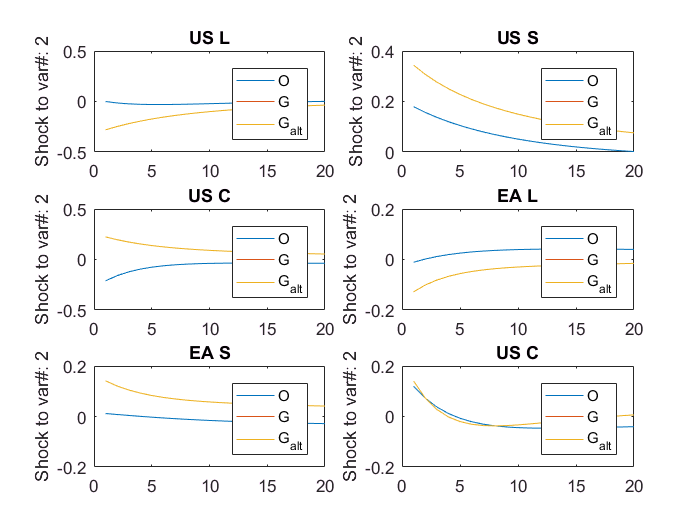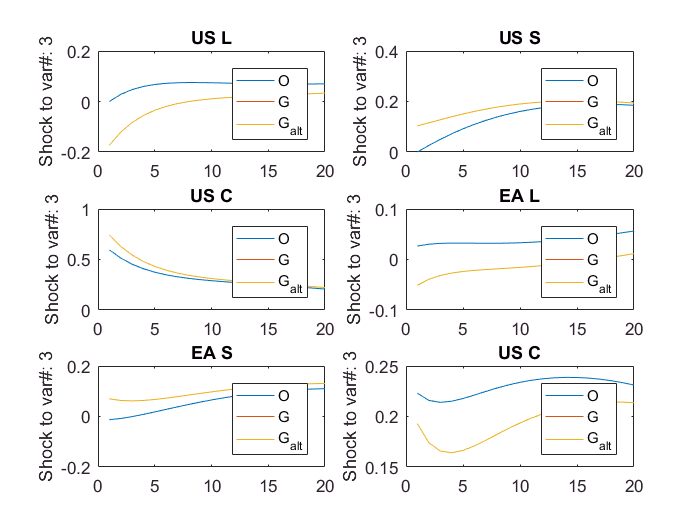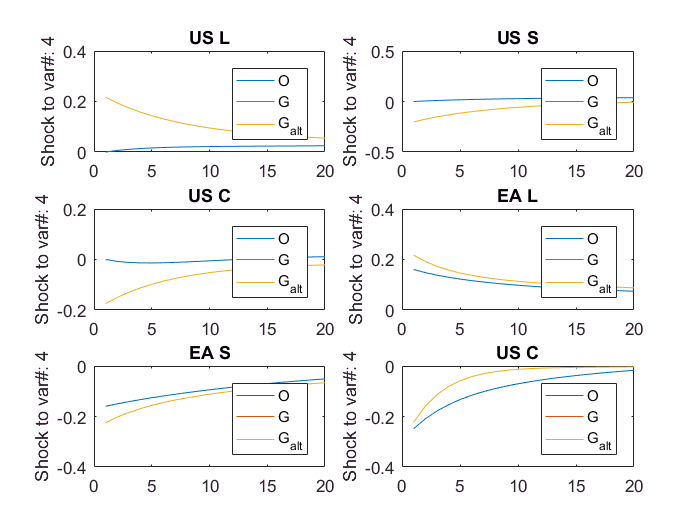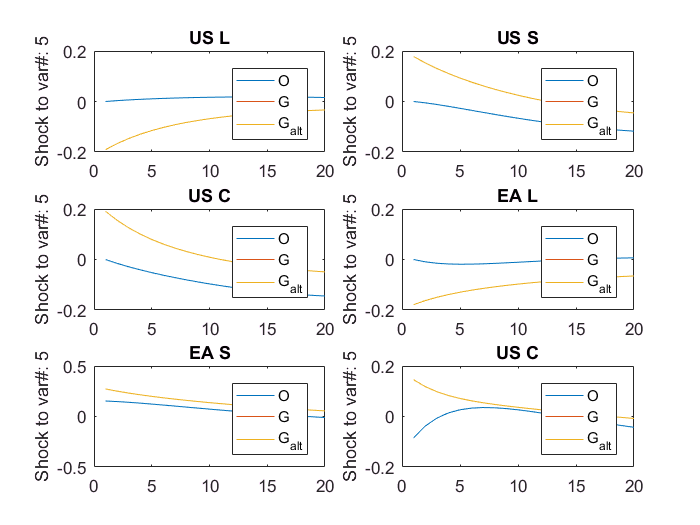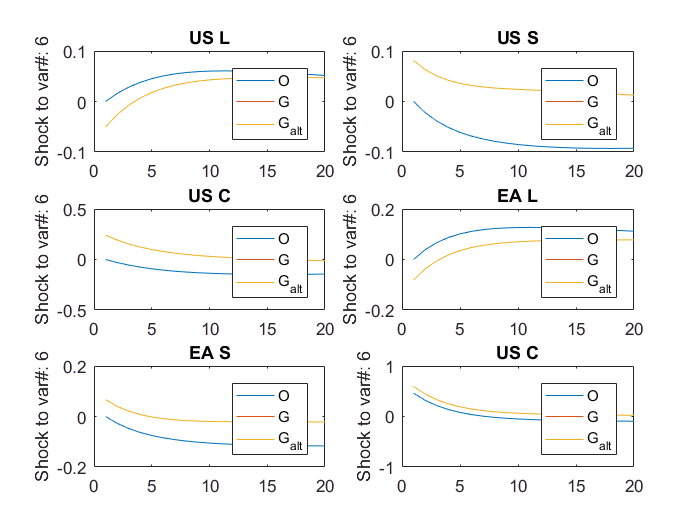It is not the purpose of this script to make an economic interpretation of the generated IRFs, but to illustrate that that the difference between a conditional and unconditional projection of the VAR model can be used to find the generalised impulse response functions. The plots above show this: the GIRFs from the Peseran and Shin expressions and the conditional projections from the model give identical results, i.e. the line plots from these calculation methodologies fall on-top of each other.